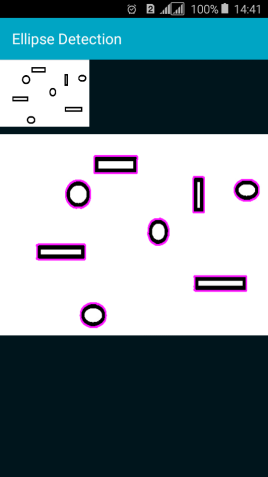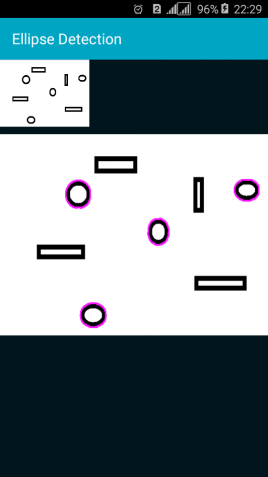# Android QuestionEllipse detection in an image with OpenCV

#### Toky Olivier

##### Active Member
Hello all,
I tried to detect circles/ellipse as done here: https://stackoverflow.com/questions/26087035/detecting-circles-in-binary-image/26125304#26125304 with this good very library: OpenCV (wrapped by @JordiCP: ) but I cannot get the perimeter of each contour. Areas, I can get them.
The function arcLength exists but it requested OCVMatOfPoint2f for the first argument. It seems that contours.Get(i) return a OCVMatOfPoint not OCVMatOfPoint2f. So I cannot get it working if someone can help.

My Code is:
B4X:
``````Sub ProcessImage
srcMat.copyTo( tmpMat)                                                        ' Clone source
dstBitmap.InitializeMutable(tmpMat.size.width,tmpMat.size.height)

Dim edge As OCVMat
Dim contours As List
Dim hierarchy As OCVMat
Dim offset As OCVPoint
Dim i As Int
mImgProc.cvtColor1(tmpMat,tmpMat,mImgProc.COLOR_RGBA2GRAY)
mImgProc.Canny3(tmpMat,edge,50,150)
offset.Initialize(0, 0)
contours.Initialize
mImgProc.findContours(edge, contours, hierarchy, mImgProc.RETR_EXTERNAL, mImgProc.CHAIN_APPROX_NONE, offset)
Dim myColorScalar As OCVScalar
myColorScalar.Initialize3(0,0,255)
For i=0 To contours.Size-1
'Dim curve As OCVMatOfPoint2f
'Dim mat As OCVMatOfPoint = contours.Get(i)
'curve.Initialize2(mat)
Dim p As Double = 0 'mImgProc.arcLength(contours.Get(i), False)
Dim a As Double = mImgProc.contourArea(contours.Get(i), False)
Log("area: " & a & " perimeter: " & p)
'If Abs(1-p*p/(4*3.1413*a))<0.2 Then
mImgProc.drawContours1(srcMat, contours, i, myColorScalar, 2)
'End If
Next

mUtils.matToBitmap1(srcMat,dstBitmap)
dstIV.Bitmap = dstBitmap

End Sub``````

Objective:It can detect all contours as you can see here:Thank you so much

#### Attachments

• 17.7 KB Views: 76
Last edited:

#### Toky Olivier

##### Active Member
Finally I solved it:
B4X:
``````Sub ProcessImage
Dim edge As OCVMat
Dim contours As List
Dim hierarchy As OCVMat
Dim offset As OCVPoint
Dim i As Int
Dim p As Double, a As Double
srcMat.copyTo( tmpMat)                                                        ' Clone source
dstBitmap.InitializeMutable(tmpMat.size.width,tmpMat.size.height)

mImgProc.cvtColor1(tmpMat,tmpMat,mImgProc.COLOR_RGBA2GRAY)
mImgProc.Canny3(tmpMat,edge,50,150)
offset.Initialize(0, 0)
contours.Initialize
mImgProc.findContours(edge, contours, hierarchy, mImgProc.RETR_EXTERNAL, mImgProc.CHAIN_APPROX_NONE, offset)
Dim myColorScalar As OCVScalar
myColorScalar.Initialize3(255,0,255)
For i=0 To contours.Size-1
Dim mat As OCVMatOfPoint = contours.Get(i)
Dim pnt() As OCVPoint = mat.toArray
Dim mat2 As OCVMatOfPoint2f
mat2.Initialize3(pnt)
p = mImgProc.arcLength(mat2, False)
a = mImgProc.contourArea(contours.Get(i), False)
Log("area: " & a & " perimeter: " & p)
If Abs(1-p*p/(4*3.1413*a))<0.2 Then
mImgProc.drawContours1(srcMat, contours, i, myColorScalar, 2)
End If
Next

mUtils.matToBitmap1(srcMat,dstBitmap)
dstIV.Bitmap = dstBitmap
End Sub``````
Screenshot:Attached the project:

#### Attachments

• 17.7 KB Views: 88